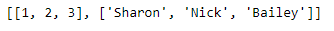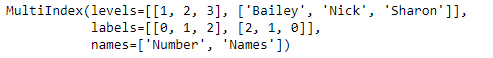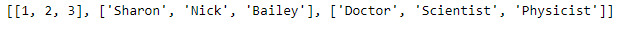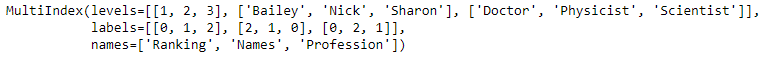# Python | Pandas MultiIndex.from_arrays()

Python is a great language for doing data analysis, primarily because of the fantastic ecosystem of data-centric python packages. Pandas is one of those packages and makes importing and analyzing data much easier.

Pandas` MultiIndex.from_arrays()` function is used to convert arrays into MultiIndex. It is one of the several ways in which we construct a MultiIndex.

Syntax: MultiIndex.from_arrays(arrays, sortorder=None, names=None)

Parameters :
arrays : Each array-like gives one level’s value for each data point. len(arrays) is the number of levels
sortorder : Level of sortedness (must be lexicographically sorted by that level)

Returns: index : MultiIndex

Example #1: Use `MultiIndex.from_arrays()` function to construct a MultiIndex from arrays.

 `# importing pandas as pd ` `import` `pandas as pd ` ` `  `# Creating the array ` `array ``=``[[``1``, ``2``, ``3``], [``'Sharon'``, ``'Nick'``, ``'Bailey'``]] ` ` `  `# Print the array ` `print``(array) `

Output :Now let’s create the MultiIndex using this array

 `# Creating the MultiIndex ` `midx ``=` `pd.MultiIndex.from_arrays(array, ` `            ``names ``=``(``'Number'``, ``'Names'``)) ` ` `  `# Print the MultiIndex ` `print``(midx) `

Output :As we can see in the output, the function has created a MultiIndex object using the arrays.

Example #2: Use `MultiIndex.from_arrays()` function to construct a MultiIndex from arrays.

 `# importing pandas as pd ` `import` `pandas as pd ` ` `  `# Creating the array ` `array ``=``[[``1``, ``2``, ``3``], [``'Sharon'``, ``'Nick'``, ``'Bailey'``],  ` `          ``[``'Doctor'``, ``'Scientist'``, ``'Physicist'``]] ` ` `  `# Print the array ` `print``(array) `

Output :Now let’s create the MultiIndex using this array

 `# Creating the MultiIndex ` `midx ``=` `pd.MultiIndex.from_arrays(array,  ` `   ``names ``=``(``'Ranking'``, ``'Names'``, ``'Profession'``)) ` ` `  `# Print the MultiIndex ` `print``(midx) `

Output :As we can see in the output, the function has created a MultiIndex using the passed arrays.

My Personal Notes arrow_drop_upCheck out this Author's contributed articles.

If you like GeeksforGeeks and would like to contribute, you can also write an article using contribute.geeksforgeeks.org or mail your article to contribute@geeksforgeeks.org. See your article appearing on the GeeksforGeeks main page and help other Geeks.

Please Improve this article if you find anything incorrect by clicking on the "Improve Article" button below.

Article Tags :

Be the First to upvote.

Please write to us at contribute@geeksforgeeks.org to report any issue with the above content.